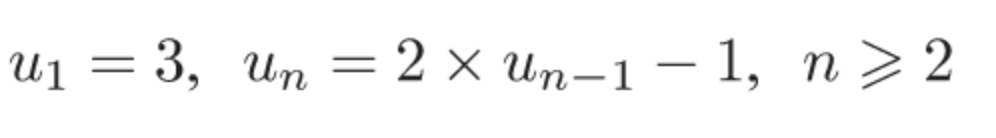# = 2 x un-1 - 1, n> 2- 3, Un

Question

Find the first four terms of the sequence with a recursive formula.help_outlineImage Transcriptionclose= 2 x un-1 - 1, n> 2 - 3, Un fullscreen
check_circleExpert Solution
Step 1

We have a recursive formula here. In a recursive formula, every subsequent term is derived from the previous term. In our  case the general recursive formula is:

un = 2 x un-1 – 1 for n ≥ 2

and u1 = 3

Step 2

We have to find the first four terms, i.e u1, u2, uand u4

Put n = 1, u1 = 3

Put n  = 2, u2 =  2 x u1 - 1 = 2 x 3 - 1 = 5

Step 3

Put n = 3, u3 = 2 x u2 - 1 = 2 x 5 - 1 = 9

Put n = 4, u4 = 2 x u...

### Want to see the full answer?

See Solution

#### Want to see this answer and more?

Solutions are written by subject experts who are available 24/7. Questions are typically answered within 1 hour*

See Solution
*Response times may vary by subject and question
Tagged in

### Functions